x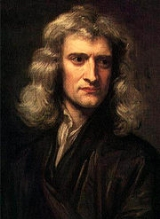MassOverview

Mass can be defined as a quantitive measure of the resistance an object has to change in its velocity.
In physics
Physics
Physics is a natural science that involves the study of matter and its motion through spacetime, along with related concepts such as energy and force. More broadly, it is the general analysis of nature, conducted in order to understand how the universe behaves.Physics is one of the oldest academic...

, mass (from ) commonly refers to any of the following three properties of matter
Matter
Matter is a general term for the substance of which all physical objects consist. Typically, matter includes atoms and other particles which have mass. A common way of defining matter is as anything that has mass and occupies volume...

, which have been shown experimentally to be equivalent:
• inertial mass
• active gravitational mass
• passive gravitational mass

Mass must be distinguished from matter in physics, because matter
Matter
Matter is a general term for the substance of which all physical objects consist. Typically, matter includes atoms and other particles which have mass. A common way of defining matter is as anything that has mass and occupies volume...

is a poorly-defined concept, and although all types of agreed-upon matter
Matter
Matter is a general term for the substance of which all physical objects consist. Typically, matter includes atoms and other particles which have mass. A common way of defining matter is as anything that has mass and occupies volume...

exhibit mass, it is also the case that many types of energy
Energy
In physics, energy is an indirectly observed quantity. It is often understood as the ability a physical system has to do work on other physical systems...

which are not matter—such as potential energy
Potential energy
In physics, potential energy is the energy stored in a body or in a system due to its position in a force field or due to its configuration. The SI unit of measure for energy and work is the Joule...

, kinetic energy
Kinetic energy
The kinetic energy of an object is the energy which it possesses due to its motion.It is defined as the work needed to accelerate a body of a given mass from rest to its stated velocity. Having gained this energy during its acceleration, the body maintains this kinetic energy unless its speed changes...

, and trapped electromagnetic radiation
Electromagnetic radiation
Electromagnetic radiation is a form of energy that exhibits wave-like behavior as it travels through space...

(photon
Photon
In physics, a photon is an elementary particle, the quantum of the electromagnetic interaction and the basic unit of light and all other forms of electromagnetic radiation. It is also the force carrier for the electromagnetic force...

s)—also exhibit mass.Unanswered QuestionsHow do you define mass and weight?Encyclopedia
Mass can be defined as a quantitive measure of the resistance an object has to change in its velocity.
In physics
Physics
Physics is a natural science that involves the study of matter and its motion through spacetime, along with related concepts such as energy and force. More broadly, it is the general analysis of nature, conducted in order to understand how the universe behaves.Physics is one of the oldest academic...

, mass (from ) commonly refers to any of the following three properties of matter
Matter
Matter is a general term for the substance of which all physical objects consist. Typically, matter includes atoms and other particles which have mass. A common way of defining matter is as anything that has mass and occupies volume...

, which have been shown experimentally to be equivalent:
• inertial mass
• active gravitational mass
• passive gravitational mass

Mass must be distinguished from matter in physics, because matter
Matter
Matter is a general term for the substance of which all physical objects consist. Typically, matter includes atoms and other particles which have mass. A common way of defining matter is as anything that has mass and occupies volume...

is a poorly-defined concept, and although all types of agreed-upon matter
Matter
Matter is a general term for the substance of which all physical objects consist. Typically, matter includes atoms and other particles which have mass. A common way of defining matter is as anything that has mass and occupies volume...

exhibit mass, it is also the case that many types of energy
Energy
In physics, energy is an indirectly observed quantity. It is often understood as the ability a physical system has to do work on other physical systems...

which are not matter—such as potential energy
Potential energy
In physics, potential energy is the energy stored in a body or in a system due to its position in a force field or due to its configuration. The SI unit of measure for energy and work is the Joule...

, kinetic energy
Kinetic energy
The kinetic energy of an object is the energy which it possesses due to its motion.It is defined as the work needed to accelerate a body of a given mass from rest to its stated velocity. Having gained this energy during its acceleration, the body maintains this kinetic energy unless its speed changes...

, and trapped electromagnetic radiation
Electromagnetic radiation
Electromagnetic radiation is a form of energy that exhibits wave-like behavior as it travels through space...

(photon
Photon
In physics, a photon is an elementary particle, the quantum of the electromagnetic interaction and the basic unit of light and all other forms of electromagnetic radiation. It is also the force carrier for the electromagnetic force...

s)—also exhibit mass. Thus, all matter has the property of mass, but not all mass is associated with identifiable matter.

In everyday usage, "mass" is often used interchangeably with weight
Weight
In science and engineering, the weight of an object is the force on the object due to gravity. Its magnitude , often denoted by an italic letter W, is the product of the mass m of the object and the magnitude of the local gravitational acceleration g; thus:...

, and the units of weight are often taken to be kilogram
Kilogram
The kilogram or kilogramme , also known as the kilo, is the base unit of mass in the International System of Units and is defined as being equal to the mass of the International Prototype Kilogram , which is almost exactly equal to the mass of one liter of water...

s (for instance, a person may state that their weight is 75kg). In scientific use, however, the two terms refer to different
Mass versus weight
In everyday usage, the mass of an object is often referred to as its weight though these are in fact different concepts and quantities. In scientific contexts, mass refers loosely to the amount of "matter" in an object , whereas weight refers to the force experienced by an object due to gravity...

, yet related, properties of matter. Weight can be zero if no gravitational force is acting but mass can never be zero.

The inertial mass of an object determines its acceleration
Acceleration
In physics, acceleration is the rate of change of velocity with time. In one dimension, acceleration is the rate at which something speeds up or slows down. However, since velocity is a vector, acceleration describes the rate of change of both the magnitude and the direction of velocity. ...

in the presence of an applied force. According to Newton's second law of motion, if a body of fixed mass M is subjected to a force F, its acceleration α is given by F/M.

A body's mass also determines the degree to which it generates or is affected by a gravitational field
Gravitational field
The gravitational field is a model used in physics to explain the existence of gravity. In its original concept, gravity was a force between point masses...

. If a first body of mass MA is placed at a distance r from a second body of mass MB, each body experiences an attractive force F whose magnitude is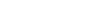where G is the universal constant of gravitation
Gravitational constant
The gravitational constant, denoted G, is an empirical physical constant involved in the calculation of the gravitational attraction between objects with mass. It appears in Newton's law of universal gravitation and in Einstein's theory of general relativity. It is also known as the universal...

, equal to . This is sometimes referred to as gravitational mass (when a distinction is necessary, M is used to denote the active gravitational mass and m the passive gravitational mass). Repeated experiments since the 17th century have demonstrated that inertial and gravitational mass are equivalent; this is entailed in the equivalence principle
Equivalence principle
In the physics of general relativity, the equivalence principle is any of several related concepts dealing with the equivalence of gravitational and inertial mass, and to Albert Einstein's assertion that the gravitational "force" as experienced locally while standing on a massive body is actually...

of general relativity
General relativity
General relativity or the general theory of relativity is the geometric theory of gravitation published by Albert Einstein in 1916. It is the current description of gravitation in modern physics...

.

Special relativity
Special relativity
Special relativity is the physical theory of measurement in an inertial frame of reference proposed in 1905 by Albert Einstein in the paper "On the Electrodynamics of Moving Bodies".It generalizes Galileo's...

shows that rest mass (or invariant mass
Invariant mass
The invariant mass, rest mass, intrinsic mass, proper mass or just mass is a characteristic of the total energy and momentum of an object or a system of objects that is the same in all frames of reference related by Lorentz transformations...

) and rest energy are essentially equivalent, via the well-known relationship (E=mc2
Mass-energy equivalence
In physics, mass–energy equivalence is the concept that the mass of a body is a measure of its energy content. In this concept, mass is a property of all energy, and energy is a property of all mass, and the two properties are connected by a constant...

). This same equation also connects relativistic mass and "relativistic energy" (total system energy). These are concepts that are related to their "rest" counterparts, but they do not have the same value, in systems where there is a net momentum. In order to deduce any of these four quantities from any of the others, in any system which has a net momentum, an equation that takes momentum into account is needed.

Mass (so long as the type and definition of mass is agreed upon) is a conserved quantity over time. From the viewpoint of any single unaccelerated observer, mass can neither be created or destroyed, and special relativity
Special relativity
Special relativity is the physical theory of measurement in an inertial frame of reference proposed in 1905 by Albert Einstein in the paper "On the Electrodynamics of Moving Bodies".It generalizes Galileo's...

does not change this understanding (though different observers may not agree on how much mass is present, all agree that the amount does not change over time). However, relativity adds the fact that all types of energy have an associated mass, and this mass is added to systems when energy is added, and the associated mass is subtracted from systems when the energy leaves. In such cases, the energy leaving or entering the system carries the added or missing mass with it, since this energy itself has mass. Thus, mass remains conserved when the location of all mass is taken into account.

On the surface of the Earth, the weight W of an object is related to its mass m by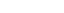where g is the Earth's gravitational field strength
Earth's gravity
The gravity of Earth, denoted g, refers to the acceleration that the Earth imparts to objects on or near its surface. In SI units this acceleration is measured in metres per second per second or equivalently in newtons per kilogram...

, equal to about . An object's weight depends on its environment, while its mass does not: an object with a mass of 50 kilogram
Kilogram
The kilogram or kilogramme , also known as the kilo, is the base unit of mass in the International System of Units and is defined as being equal to the mass of the International Prototype Kilogram , which is almost exactly equal to the mass of one liter of water...

s weighs 491 newtons on the surface of the Earth; on the surface of the Moon
Moon
The Moon is Earth's only known natural satellite,There are a number of near-Earth asteroids including 3753 Cruithne that are co-orbital with Earth: their orbits bring them close to Earth for periods of time but then alter in the long term . These are quasi-satellites and not true moons. For more...

, the same object still has a mass of 50 kilograms but weighs only 81.5 newtons.

## Units of mass

In the International System of Units
International System of Units
The International System of Units is the modern form of the metric system and is generally a system of units of measurement devised around seven base units and the convenience of the number ten. The older metric system included several groups of units...

(SI), mass is measured in kilogram
Kilogram
The kilogram or kilogramme , also known as the kilo, is the base unit of mass in the International System of Units and is defined as being equal to the mass of the International Prototype Kilogram , which is almost exactly equal to the mass of one liter of water...

s (kg). The gram
Gram
The gram is a metric system unit of mass....

(g) is of a kilogram.

Other units are accepted for use in SI:
• The tonne
Tonne
The tonne, known as the metric ton in the US , often put pleonastically as "metric tonne" to avoid confusion with ton, is a metric system unit of mass equal to 1000 kilograms. The tonne is not an International System of Units unit, but is accepted for use with the SI...

(t) is equal to 1000 kg.
• The electronvolt
Electronvolt
In physics, the electron volt is a unit of energy equal to approximately joule . By definition, it is equal to the amount of kinetic energy gained by a single unbound electron when it accelerates through an electric potential difference of one volt...

(eV) is primarily a unit of energy
Energy
In physics, energy is an indirectly observed quantity. It is often understood as the ability a physical system has to do work on other physical systems...

, but because of the mass-energy equivalence
Mass-energy equivalence
In physics, mass–energy equivalence is the concept that the mass of a body is a measure of its energy content. In this concept, mass is a property of all energy, and energy is a property of all mass, and the two properties are connected by a constant...

it can also function as a unit of mass. In this context it is denoted eV/c2, or simply as eV. The electronvolt is common in particle physics
Particle physics
Particle physics is a branch of physics that studies the existence and interactions of particles that are the constituents of what is usually referred to as matter or radiation. In current understanding, particles are excitations of quantum fields and interact following their dynamics...

.
• The atomic mass unit
Atomic mass unit
The unified atomic mass unit or dalton is a unit that is used for indicating mass on an atomic or molecular scale. It is defined as one twelfth of the rest mass of an unbound neutral atom of carbon-12 in its nuclear and electronic ground state, and has a value of...

(u) is defined so that a single carbon-12
Carbon-12
Carbon-12 is the more abundant of the two stable isotopes of the element carbon, accounting for 98.89% of carbon; it contains 6 protons, 6 neutrons, and 6 electrons....

atom has a mass of 12 u; 1 u is approximately .Since the Avogadro constant NA is defined as the number of atoms in 12 g of carbon-12, it follows that 1 u is exactly 1/(103 NA) kg. The atomic mass unit is convenient for expressing the masses of atoms and molecules.

Outside the SI system, a variety of different mass units are used, depending on context, such as the slug (sl), the pound
Pound (mass)
The pound or pound-mass is a unit of mass used in the Imperial, United States customary and other systems of measurement...

(lb), the Planck mass (mP), and the solar mass
Solar mass
The solar mass , , is a standard unit of mass in astronomy, used to indicate the masses of other stars and galaxies...

In normal situations, the weight of an object is proportional to its mass, which usually makes it unproblematic to use the same unit for both concepts. However, the distinction between mass and weight
Mass versus weight
In everyday usage, the mass of an object is often referred to as its weight though these are in fact different concepts and quantities. In scientific contexts, mass refers loosely to the amount of "matter" in an object , whereas weight refers to the force experienced by an object due to gravity...

becomes important for measurements with a precision better than a few percent (because of slight differences in the strength of the Earth's gravitational field at different places), and for places far from the surface of the Earth, such as in space or on other planets.

A mass can sometimes be expressed in terms of length. The mass of a very small particle may be identified with its inverse Compton wavelength
Compton wavelength
The Compton wavelength is a quantum mechanical property of a particle. It was introduced by Arthur Compton in his explanation of the scattering of photons by electrons...

. The mass of a very large star or black hole
Black hole
A black hole is a region of spacetime from which nothing, not even light, can escape. The theory of general relativity predicts that a sufficiently compact mass will deform spacetime to form a black hole. Around a black hole there is a mathematically defined surface called an event horizon that...

may be identified with its Schwarzschild radius
Schwarzschild radius
The Schwarzschild radius is the distance from the center of an object such that, if all the mass of the object were compressed within that sphere, the escape speed from the surface would equal the speed of light...

.

## Summary of mass concepts and formalisms

In classical mechanics
Classical mechanics
In physics, classical mechanics is one of the two major sub-fields of mechanics, which is concerned with the set of physical laws describing the motion of bodies under the action of a system of forces...

, mass has a central role in determining the behavior of bodies. Newton's second law relates the force
Force
In physics, a force is any influence that causes an object to undergo a change in speed, a change in direction, or a change in shape. In other words, a force is that which can cause an object with mass to change its velocity , i.e., to accelerate, or which can cause a flexible object to deform...

F exerted in a body of mass M to the body's acceleration
Acceleration
In physics, acceleration is the rate of change of velocity with time. In one dimension, acceleration is the rate at which something speeds up or slows down. However, since velocity is a vector, acceleration describes the rate of change of both the magnitude and the direction of velocity. ...

α: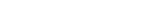.
Additionally, mass relates a body's momentum
Momentum
In classical mechanics, linear momentum or translational momentum is the product of the mass and velocity of an object...

p to its velocity
Velocity
In physics, velocity is speed in a given direction. Speed describes only how fast an object is moving, whereas velocity gives both the speed and direction of the object's motion. To have a constant velocity, an object must have a constant speed and motion in a constant direction. Constant ...

v: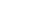,
and the body's kinetic energy
Kinetic energy
The kinetic energy of an object is the energy which it possesses due to its motion.It is defined as the work needed to accelerate a body of a given mass from rest to its stated velocity. Having gained this energy during its acceleration, the body maintains this kinetic energy unless its speed changes...

K to its velocity: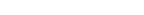.

In special relativity
Special relativity
Special relativity is the physical theory of measurement in an inertial frame of reference proposed in 1905 by Albert Einstein in the paper "On the Electrodynamics of Moving Bodies".It generalizes Galileo's...

, relativistic mass is a formalism which accounts for relativistic effects by having the mass increase with velocity.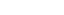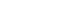Since energy is dependent on reference frame (upon the observer) it is convenient to formulate the equations of physics in a way such that mass values are invariant (do not change) between observers, and so the equations are independent of the observer. For a single particle, this quantity is the rest mass; for a system of bound or unbound particles, this quantity is the invariant mass. The invariant mass M of a body is related to its energy E and the magnitude of its momentum p by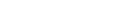where c is the speed of light
Speed of light
The speed of light in vacuum, usually denoted by c, is a physical constant important in many areas of physics. Its value is 299,792,458 metres per second, a figure that is exact since the length of the metre is defined from this constant and the international standard for time...

.

## Summary of mass related phenomena

In physical science
Physical science
Physical science is an encompassing term for the branches of natural science and science that study non-living systems, in contrast to the life sciences...

, one may distinguish conceptually between at least seven attributes of mass, or seven physical phenomena that can be explained using the concept of mass:
• The amount of matter
Matter
Matter is a general term for the substance of which all physical objects consist. Typically, matter includes atoms and other particles which have mass. A common way of defining matter is as anything that has mass and occupies volume...

in certain types of samples can be exactly determined through electrodeposition
Electrophoretic deposition
Electrophoretic deposition , is a term for a broad range of industrial processes which includes electrocoating, e-coating, cathodic electrodeposition, and electrophoretic coating, or electrophoretic painting...

or other precise processes. The mass of an exact sample is determined in part by the number and type of atoms or molecules it contains, and in part by the energy involved in binding it together (which contributes a negative "missing mass," or mass deficit).

• Inertial mass is a measure of an object's resistance to changing its state of motion when a force
Force
In physics, a force is any influence that causes an object to undergo a change in speed, a change in direction, or a change in shape. In other words, a force is that which can cause an object with mass to change its velocity , i.e., to accelerate, or which can cause a flexible object to deform...

is applied. It is determined by applying a force to an object and measuring the acceleration that results from that force. An object with small inertial mass will accelerate more than an object with large inertial mass when acted upon by the same force. One says the body of greater mass has greater inertia
Inertia
Inertia is the resistance of any physical object to a change in its state of motion or rest, or the tendency of an object to resist any change in its motion. It is proportional to an object's mass. The principle of inertia is one of the fundamental principles of classical physics which are used to...

.

• Active gravitational mass is a measure of the strength of an object’s gravitational flux
Gauss' law for gravity
In physics, Gauss's law for gravity, also known as Gauss's flux theorem for gravity, is a law of physics which is essentially equivalent to Newton's law of universal gravitation...

(gravitational flux is equal to the surface integral of gravitational field over an enclosing surface). Gravitational field can be measured by allowing a small ‘test object’ to freely fall and measuring its free-fall
Free-fall
Free fall is any motion of a body where gravity is the only force acting upon it, at least initially. These conditions produce an inertial trajectory so long as gravity remains the only force. Since this definition does not specify velocity, it also applies to objects initially moving upward...

acceleration. For example, an object in free-fall near the Moon
Moon
The Moon is Earth's only known natural satellite,There are a number of near-Earth asteroids including 3753 Cruithne that are co-orbital with Earth: their orbits bring them close to Earth for periods of time but then alter in the long term . These are quasi-satellites and not true moons. For more...

will experience less gravitational field, and hence accelerate slower than the same object would if it were in free-fall near the Earth. The gravitational field near the Moon is weaker because the Moon has less active gravitational mass.

• Passive gravitational mass is a measure of the strength of an object's interaction with a gravitational field
Gravitational field
The gravitational field is a model used in physics to explain the existence of gravity. In its original concept, gravity was a force between point masses...

. Passive gravitational mass is determined by dividing an object’s weight by its free-fall acceleration. Two objects within the same gravitational field will experience the same acceleration; however, the object with a smaller passive gravitational mass will experience a smaller force (less weight) than the object with a larger passive gravitational mass.

• Energy also has mass according to the principle of mass–energy equivalence. This equivalence is exemplified in a large number of physical processes including pair production
Pair production
Pair production refers to the creation of an elementary particle and its antiparticle, usually from a photon . For example an electron and its antiparticle, the positron, may be created...

, nuclear fusion
Nuclear fusion
Nuclear fusion is the process by which two or more atomic nuclei join together, or "fuse", to form a single heavier nucleus. This is usually accompanied by the release or absorption of large quantities of energy...

, and the gravitational bending of light
Gravitational lens
A gravitational lens refers to a distribution of matter between a distant source and an observer, that is capable of bending the light from the source, as it travels towards the observer...

. Pair production and nuclear fusion are processes through which measurable amounts of mass and energy are converted into each other. In the gravitational bending of light, photons of pure energy are shown to exhibit a behavior similar to passive gravitational mass.

• Curvature of spacetime
Spacetime
In physics, spacetime is any mathematical model that combines space and time into a single continuum. Spacetime is usually interpreted with space as being three-dimensional and time playing the role of a fourth dimension that is of a different sort from the spatial dimensions...

is a relativistic manifestation of the existence of mass. Curvature
Curvature
In mathematics, curvature refers to any of a number of loosely related concepts in different areas of geometry. Intuitively, curvature is the amount by which a geometric object deviates from being flat, or straight in the case of a line, but this is defined in different ways depending on the context...

is extremely weak and difficult to measure. For this reason, curvature wasn’t discovered until after it was predicted by Einstein’s theory of general relativity. Extremely precise atomic clocks on the surface of the earth, for example, are found to measure less time (run slower) than similar clocks in space. This difference in elapsed time is a form of curvature called gravitational time dilation
Gravitational time dilation
Gravitational time dilation is the effect of time passing at different rates in regions of different gravitational potential; the lower the gravitational potential, the more slowly time passes...

. Other forms of curvature have been measured using the Gravity Probe B
Gravity Probe B
Gravity Probe B is a satellite-based mission which launched on 20 April 2004 on a Delta II rocket. The spaceflight phase lasted until 2005; its aim was to measure spacetime curvature near Earth, and thereby the stress–energy tensor in and near Earth...

satellite.

• Quantum mass manifests itself as a difference between an object’s quantum frequency
Frequency
Frequency is the number of occurrences of a repeating event per unit time. It is also referred to as temporal frequency.The period is the duration of one cycle in a repeating event, so the period is the reciprocal of the frequency...

and its wave number. The quantum mass of an electron, the Compton wavelength
Compton wavelength
The Compton wavelength is a quantum mechanical property of a particle. It was introduced by Arthur Compton in his explanation of the scattering of photons by electrons...

, can be determined through various forms of spectroscopy
Spectroscopy
Spectroscopy is the study of the interaction between matter and radiated energy. Historically, spectroscopy originated through the study of visible light dispersed according to its wavelength, e.g., by a prism. Later the concept was expanded greatly to comprise any interaction with radiative...

and is closely related to the Rydberg constant
Rydberg constant
The Rydberg constant, symbol R∞, named after the Swedish physicist Johannes Rydberg, is a physical constant relating to atomic spectra in the science of spectroscopy. Rydberg initially determined its value empirically from spectroscopy, but Niels Bohr later showed that its value could be calculated...

, the Bohr radius
Bohr radius
The Bohr radius is a physical constant, approximately equal to the most probable distance between the proton and electron in a hydrogen atom in its ground state. It is named after Niels Bohr, due to its role in the Bohr model of an atom...

, and the classical electron radius
Classical electron radius
The classical electron radius, also known as the Lorentz radius or the Thomson scattering length, is based on a classical relativistic model of the electron...

. The quantum mass of larger objects can be directly measured using a watt balance
Watt balance
The watt balance is an experimental electromechanical weight measuring instrument that measures the weight of a test object very precisely by the strength of an electric current and a voltage. It is being developed as a metrological instrument that may one day provide a definition of the kilogram...

.

Inertial mass, gravitational mass, and the various other mass-related phenomena are conceptually distinct. However, every experiment to date has shown these values to be proportional
Proportionality (mathematics)
In mathematics, two variable quantities are proportional if one of them is always the product of the other and a constant quantity, called the coefficient of proportionality or proportionality constant. In other words, are proportional if the ratio \tfrac yx is constant. We also say that one...

, and this proportionality gives rise to the abstract concept of mass. If, in some future experiment, one of the mass-related phenomena is shown to not be proportional to the others, then that specific phenomenon will no longer be considered a part of the abstract concept of mass.

## Weight and amount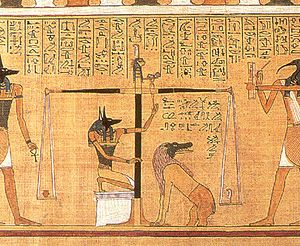Weight
Weight
In science and engineering, the weight of an object is the force on the object due to gravity. Its magnitude , often denoted by an italic letter W, is the product of the mass m of the object and the magnitude of the local gravitational acceleration g; thus:...

, by definition, is a measure of the force which must be applied to support an object (i.e. hold it at rest) in a gravitational field. The Earth’s gravitational field causes items near the Earth to have weight. Typically, gravitational fields change only slightly over short distances, and the Earth’s field is nearly uniform at all locations on the Earth’s surface; therefore, an object’s weight changes only slightly when it is moved from one location to another, and these small changes went unnoticed through much of history. This may have given early humans the impression that weight is an unchanging, fundamental property of objects in the material world.

In the Egyptian religious illustration
Book of the Dead
The Book of the Dead is the modern name of an ancient Egyptian funerary text, used from the beginning of the New Kingdom to around 50 BC. The original Egyptian name for the text, transliterated rw nw prt m hrw is translated as "Book of Coming Forth by Day". Another translation would be "Book of...

to the right, Anubis is using a balance scale to weigh the heart of Hunefer
Hunefer
Hunefer was a scribe during the 19th Dynasty. His copy of the Egyptian funerary Book of the Dead, along with the Papyrus of Ani, represent classical examples of these documents. He was "Scribe of Divine Offerings", "Overseer of Royal Cattle", and steward of Pharaoh Seti I.-External links:*...

. A balance scale balances the force of one object’s weight against the force of another object’s weight. The two sides of a balance scale are close enough that the objects experience similar gravitational fields. Hence, if they have similar masses then their weights will also be similar. This allows the scale, by comparing weights, to also compare masses, and gives it the distinction of being one of the oldest known devices capable of measuring mass.

The concept of amount is very old and predates recorded history, so any description of the early development of this concept is speculative in nature. However, one might reasonably assume that humans, at some early era, realized that the weight of a collection of similar objects was directly proportional
Proportionality (mathematics)
In mathematics, two variable quantities are proportional if one of them is always the product of the other and a constant quantity, called the coefficient of proportionality or proportionality constant. In other words, are proportional if the ratio \tfrac yx is constant. We also say that one...

to the number of objects in the collection: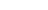,

where w is the weight of the collection of similar objects and n is the number of objects in the collection. Proportionality, by definition, implies that two values have a constant ratio
Ratio
In mathematics, a ratio is a relationship between two numbers of the same kind , usually expressed as "a to b" or a:b, sometimes expressed arithmetically as a dimensionless quotient of the two which explicitly indicates how many times the first number contains the second In mathematics, a ratio is...

: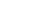, or equivalently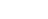.

Consequently, historical weight standards were often defined in terms of amounts. The Romans, for example, used the carob seed (carat or siliqua
Siliqua
The siliqua is the modern name given to small, thin, Roman silver coins produced from 4th century and later. When the coins were in circulation, the Latin word siliqua was a unit of weight defined as one-twentyfourth of the weight of a Roman solidus .The term siliqua comes from the siliqua graeca,...

) as a measurement standard. If an object’s weight was equivalent to 1728 carob seeds, then the object was said to weigh one Roman pound. If, on the other hand, the object’s weight was equivalent to 144 carob seeds
Ancient Roman units of measurement
The ancient Roman units of measurement were built on the Hellenic system with Egyptian, Hebrew, and Mesopotamian influences. The Roman units were comparatively consistent and well documented.-Length:Notes...

then the object was said to weigh one Roman ounce (uncia). The Roman pound and ounce were both defined in terms of different sized collections of the same common mass standard, the carob seed. The ratio of a Roman ounce (144 carob seeds) to a Roman pound (1728 carob seeds) was: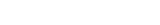.

This example illustrates a common occurrence in physical science: when values are related through simple fractions, there is a good possibility that the values stem from a common source.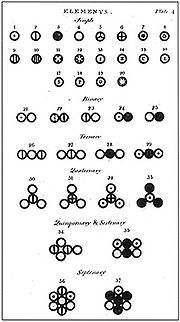The name atom
Atom
The atom is a basic unit of matter that consists of a dense central nucleus surrounded by a cloud of negatively charged electrons. The atomic nucleus contains a mix of positively charged protons and electrically neutral neutrons...

comes from the Greek
Greek language
Greek is an independent branch of the Indo-European family of languages. Native to the southern Balkans, it has the longest documented history of any Indo-European language, spanning 34 centuries of written records. Its writing system has been the Greek alphabet for the majority of its history;...

ἄτομος/átomos, α-τεμνω, which means uncuttable, something that cannot be divided further. The philosophical concept that matter might be composed of discrete units that cannot be further divided has been around for millennia. However, empirical proof and the universal acceptance of the existence of atoms didn’t occur until the early 20th century.

As the science of chemistry matured, experimental evidence for the existence of atoms came from the law of multiple proportions
Law of multiple proportions
In chemistry, the law of multiple proportions is one of the basic laws of stoichiometry, alongside the law of definite proportions. It is sometimes called Dalton's Law after its discoverer, the English chemist John Dalton.The statement of the law is:...

. When two or more elements combined to form a compound, their masses are always in a fixed and definite ratio. For example, the mass ratio of nitrogen to oxygen in nitric oxide is seven eights. Ammonia has a hydrogen to nitrogen mass ratio of three fourteenths. The fact that elemental masses combined in simple fractions implies that all elemental mass stems from a common source. In principle, the atomic mass situation is analogous to the above example of Roman mass units. The Roman pound and ounce were both defined in terms of different sized collections of carob seeds, and consequently, the two mass units were related to each other through a simple fraction. Comparatively, since all of the atomic masses are related to each other through simple fractions, then perhaps the atomic masses are just different sized collections of some common fundamental mass unit.

In 1805, the chemist John Dalton published his first table of relative atomic weights, listing six elements, hydrogen
Hydrogen
Hydrogen is the chemical element with atomic number 1. It is represented by the symbol H. With an average atomic weight of , hydrogen is the lightest and most abundant chemical element, constituting roughly 75% of the Universe's chemical elemental mass. Stars in the main sequence are mainly...

, oxygen
Oxygen
Oxygen is the element with atomic number 8 and represented by the symbol O. Its name derives from the Greek roots ὀξύς and -γενής , because at the time of naming, it was mistakenly thought that all acids required oxygen in their composition...

, nitrogen
Nitrogen
Nitrogen is a chemical element that has the symbol N, atomic number of 7 and atomic mass 14.00674 u. Elemental nitrogen is a colorless, odorless, tasteless, and mostly inert diatomic gas at standard conditions, constituting 78.08% by volume of Earth's atmosphere...

, carbon
Carbon
Carbon is the chemical element with symbol C and atomic number 6. As a member of group 14 on the periodic table, it is nonmetallic and tetravalent—making four electrons available to form covalent chemical bonds...

, sulfur
Sulfur
Sulfur or sulphur is the chemical element with atomic number 16. In the periodic table it is represented by the symbol S. It is an abundant, multivalent non-metal. Under normal conditions, sulfur atoms form cyclic octatomic molecules with chemical formula S8. Elemental sulfur is a bright yellow...

, and phosphorus
Phosphorus
Phosphorus is the chemical element that has the symbol P and atomic number 15. A multivalent nonmetal of the nitrogen group, phosphorus as a mineral is almost always present in its maximally oxidized state, as inorganic phosphate rocks...

, and assigning hydrogen an atomic weight of 1. And in 1815, the chemist William Prout
William Prout
William Prout FRS was an English chemist, physician, and natural theologian. He is remembered today mainly for what is called Prout's hypothesis.-Biography:...

concluded that the hydrogen atom was in fact the fundamental mass unit from which all other atomic masses were derived.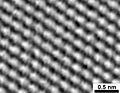If Prout's hypothesis
Prout's hypothesis
Prout's hypothesis was an early 19th century attempt to explain the existence of the various chemical elements through a hypothesis regarding the internal structure of the atom...

had proven accurate, then the abstract concept of mass, as we now know it, might never have evolved, since mass could always be defined in terms of amounts of the hydrogen atomic mass. Prout’s hypothesis; however, was found to be inaccurate in two major respects. First, further scientific advancements revealed the existence of smaller particles, such as electrons and quarks, whose masses are not related through simple fractions. And second, the elemental masses themselves were found to not be exact multiples of the hydrogen atom mass, but rather, they were near multiples. Einstein’s theory of relativity explained that when protons and neutrons come together to form an atomic nucleus
Atomic nucleus
The nucleus is the very dense region consisting of protons and neutrons at the center of an atom. It was discovered in 1911, as a result of Ernest Rutherford's interpretation of the famous 1909 Rutherford experiment performed by Hans Geiger and Ernest Marsden, under the direction of Rutherford. The...

, some of the mass of the nucleus is released in the form of binding energy
Binding energy
Binding energy is the mechanical energy required to disassemble a whole into separate parts. A bound system typically has a lower potential energy than its constituent parts; this is what keeps the system together—often this means that energy is released upon the creation of a bound state...

. The more tightly bound the nucleus, the more energy is lost during formation and this binding energy loss causes the elemental masses to not be related through simple fractions.

Hydrogen, for example, with a single proton, has an atomic weight of 1.007825 u. The most abundant isotope of iron
Isotopes of iron
Naturally occurring iron consists of four isotopes: 5.845% of 54Fe , 91.754% of 56Fe, 2.119% of 57Fe and 0.282% of 58Fe. There are 24 known radioactive isotopes and their half-lives are shown below...

has 26 protons and 30 neutrons, so one might expect its atomic weight to be 56 times that of the hydrogen atom, but in fact, its atomic weight is only 55.9383 u, which is clearly not an integer
Integer
The integers are formed by the natural numbers together with the negatives of the non-zero natural numbers .They are known as Positive and Negative Integers respectively...

multiple of 1.007825. Prout’s hypothesis was proven inaccurate in many respects, but the abstract concepts of atomic mass and amount continue to play an influential role in chemistry, and the atomic mass unit
Atomic mass unit
The unified atomic mass unit or dalton is a unit that is used for indicating mass on an atomic or molecular scale. It is defined as one twelfth of the rest mass of an unbound neutral atom of carbon-12 in its nuclear and electronic ground state, and has a value of...

continues to be the unit of choice for very small mass measurements.

When the French invented the metric system in the late 18th century, they used an amount to define their mass unit. The kilogram was originally defined to be equal in mass to the amount of pure water contained in a one-liter container. This definition, however, was inadequate for the precision requirements of modern technology, and the metric kilogram
Kilogram
The kilogram or kilogramme , also known as the kilo, is the base unit of mass in the International System of Units and is defined as being equal to the mass of the International Prototype Kilogram , which is almost exactly equal to the mass of one liter of water...

was redefined in terms of a man-made platinum-iridium bar known as the international prototype kilogram.

## Gravitational mass

Active gravitational mass is a property of the mass of an object that produces a gravitational field in the space surrounding the object, and these gravitational fields govern large-scale structures in the Universe
Universe
The Universe is commonly defined as the totality of everything that exists, including all matter and energy, the planets, stars, galaxies, and the contents of intergalactic space. Definitions and usage vary and similar terms include the cosmos, the world and nature...

. Gravitational fields hold the galaxies together. They cause clouds of gas
Gas
Gas is one of the three classical states of matter . Near absolute zero, a substance exists as a solid. As heat is added to this substance it melts into a liquid at its melting point , boils into a gas at its boiling point, and if heated high enough would enter a plasma state in which the electrons...

and dust
Dust
Dust consists of particles in the atmosphere that arise from various sources such as soil dust lifted up by wind , volcanic eruptions, and pollution...

to coalesce into stars and planets. They provide the necessary pressure for nuclear fusion
Nuclear fusion
Nuclear fusion is the process by which two or more atomic nuclei join together, or "fuse", to form a single heavier nucleus. This is usually accompanied by the release or absorption of large quantities of energy...

to occur within stars. And they determine the orbits of various objects within the Solar System
Solar System
The Solar System consists of the Sun and the astronomical objects gravitationally bound in orbit around it, all of which formed from the collapse of a giant molecular cloud approximately 4.6 billion years ago. The vast majority of the system's mass is in the Sun...

. Since gravitational effects are all around us, it is impossible to pin down the exact date when humans first discovered gravitational mass. However, it is possible to identify some of the significant steps towards our modern understanding of gravitational mass and its relationship to the other mass phenomena. Some terms associated with gravitational mass and its effects are the Gaussian gravitational constant
Gaussian gravitational constant
The Gaussian gravitational constant is an astronomical constant first proposed by German polymath Carl Friedrich Gauss in his 1809 work Theoria motus corporum coelestium in sectionibus conicis solem ambientum , although he had already used the concept to great success in predicting the...

, the standard gravitational parameter
Standard gravitational parameter
In astrodynamics, the standard gravitational parameter μ of a celestial body is the product of the gravitational constant G and the mass M of the body.\mu=GM \ The SI units of the standard gravitational parameter are m3s−2....

and the Schwarzschild radius.

### Keplerian gravitational massEnglish
name
| |The Keplerian planets
Semi-major axis
Semi-major axis
The major axis of an ellipse is its longest diameter, a line that runs through the centre and both foci, its ends being at the widest points of the shape...

Sidereal orbital period  Mass of Sun
Mercury
Mercury (planet)
Mercury is the innermost and smallest planet in the Solar System, orbiting the Sun once every 87.969 Earth days. The orbit of Mercury has the highest eccentricity of all the Solar System planets, and it has the smallest axial tilt. It completes three rotations about its axis for every two orbits...

0.387 099 AU
Astronomical unit
An astronomical unit is a unit of length equal to about or approximately the mean Earth–Sun distance....

0.240 842 sidereal year
Sidereal year
A sidereal year is the time taken by the Earth to orbit the Sun once with respect to the fixed stars. Hence it is also the time taken for the Sun to return to the same position with respect to the fixed stars after apparently travelling once around the ecliptic. It was equal to at noon 1 January...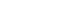Venus
Venus
Venus is the second planet from the Sun, orbiting it every 224.7 Earth days. The planet is named after Venus, the Roman goddess of love and beauty. After the Moon, it is the brightest natural object in the night sky, reaching an apparent magnitude of −4.6, bright enough to cast shadows...

0.723 332 AU 0.615 187 sidereal year
Earth
Earth
Earth is the third planet from the Sun, and the densest and fifth-largest of the eight planets in the Solar System. It is also the largest of the Solar System's four terrestrial planets...

1.000 000 AU 1.000 000 sidereal year
Mars
Mars
Mars is the fourth planet from the Sun in the Solar System. The planet is named after the Roman god of war, Mars. It is often described as the "Red Planet", as the iron oxide prevalent on its surface gives it a reddish appearance...

1.523 662 AU 1.880 816 sidereal year
Jupiter
Jupiter
Jupiter is the fifth planet from the Sun and the largest planet within the Solar System. It is a gas giant with mass one-thousandth that of the Sun but is two and a half times the mass of all the other planets in our Solar System combined. Jupiter is classified as a gas giant along with Saturn,...

5.203 363 AU 11.861 776 sidereal year
Saturn
Saturn
Saturn is the sixth planet from the Sun and the second largest planet in the Solar System, after Jupiter. Saturn is named after the Roman god Saturn, equated to the Greek Cronus , the Babylonian Ninurta and the Hindu Shani. Saturn's astronomical symbol represents the Roman god's sickle.Saturn,...

9.537 070 AU 29.456 626 sidereal year

Johannes Kepler
Johannes Kepler
Johannes Kepler was a German mathematician, astronomer and astrologer. A key figure in the 17th century scientific revolution, he is best known for his eponymous laws of planetary motion, codified by later astronomers, based on his works Astronomia nova, Harmonices Mundi, and Epitome of Copernican...

was the first to give an accurate description of the orbits of the planets, and by doing so; he was the first to describe gravitational mass. In 1600 AD, Kepler sought employment with Tycho Brahe
Tycho Brahe
Tycho Brahe , born Tyge Ottesen Brahe, was a Danish nobleman known for his accurate and comprehensive astronomical and planetary observations...

and consequently gained access to astronomical data of a higher precision than any previously available. Using Brahe’s precise observations of the planet Mars, Kepler realized that the traditional astronomical methods were inaccurate in their predictions, and he spent the next five years developing his own method for characterizing planetary motion.

In Kepler’s final planetary model, he successfully described planetary orbits as following elliptical paths with the Sun at a focal point of the ellipse. The concept of active gravitational mass is an immediate consequence of Kepler's third law of planetary motion
Kepler's laws of planetary motion
In astronomy, Kepler's laws give a description of the motion of planets around the Sun.Kepler's laws are:#The orbit of every planet is an ellipse with the Sun at one of the two foci....

. Kepler discovered that the square of the orbital period
Orbital period
The orbital period is the time taken for a given object to make one complete orbit about another object.When mentioned without further qualification in astronomy this refers to the sidereal period of an astronomical object, which is calculated with respect to the stars.There are several kinds of...

of each planet is directly proportional
Proportionality (mathematics)
In mathematics, two variable quantities are proportional if one of them is always the product of the other and a constant quantity, called the coefficient of proportionality or proportionality constant. In other words, are proportional if the ratio \tfrac yx is constant. We also say that one...

to the cube
Cube (arithmetic)
In arithmetic and algebra, the cube of a number n is its third power — the result of the number multiplying by itself three times:...

of the semi-major axis
Semi-major axis
The major axis of an ellipse is its longest diameter, a line that runs through the centre and both foci, its ends being at the widest points of the shape...

of its orbit, or equivalently, that the ratio
Ratio
In mathematics, a ratio is a relationship between two numbers of the same kind , usually expressed as "a to b" or a:b, sometimes expressed arithmetically as a dimensionless quotient of the two which explicitly indicates how many times the first number contains the second In mathematics, a ratio is...

of these two values is constant for all planets in the Solar System
Solar System
The Solar System consists of the Sun and the astronomical objects gravitationally bound in orbit around it, all of which formed from the collapse of a giant molecular cloud approximately 4.6 billion years ago. The vast majority of the system's mass is in the Sun...

. This constant ratio is a direct measure of the Sun's active gravitational mass, it has units of distance cubed per time squared, and is known as the standard gravitational parameter
Standard gravitational parameter
In astrodynamics, the standard gravitational parameter μ of a celestial body is the product of the gravitational constant G and the mass M of the body.\mu=GM \ The SI units of the standard gravitational parameter are m3s−2....

: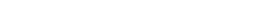English
name
| |The Galilean moons
Semi-major axis Sidereal orbital period Mass of Jupiter
Io
Io (moon)
Io ) is the innermost of the four Galilean moons of the planet Jupiter and, with a diameter of , the fourth-largest moon in the Solar System. It was named after the mythological character of Io, a priestess of Hera who became one of the lovers of Zeus....

0.002 819 AU 0.004 843 sidereal year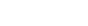Europa
Europa (moon)
Europa Slightly smaller than Earth's Moon, Europa is primarily made of silicate rock and probably has an iron core. It has a tenuous atmosphere composed primarily of oxygen. Its surface is composed of ice and is one of the smoothest in the Solar System. This surface is striated by cracks and...

0.004 486 AU 0.009 722 sidereal year
Ganymede
Ganymede (moon)
Ganymede is a satellite of Jupiter and the largest moon in the Solar System. It is the seventh moon and third Galilean satellite outward from Jupiter. Completing an orbit in roughly seven days, Ganymede participates in a 1:2:4 orbital resonance with the moons Europa and Io, respectively...

0.007 155 AU 0.019 589 sidereal year
Callisto
Callisto (moon)
Callisto named after the Greek mythological figure of Callisto) is a moon of the planet Jupiter. It was discovered in 1610 by Galileo Galilei. It is the third-largest moon in the Solar System and the second largest in the Jovian system, after Ganymede. Callisto has about 99% the diameter of the...

0.012 585 AU 0.045 694 sidereal year

In 1609, Johannes Kepler published his three rules known as Kepler's laws of planetary motion, explaining how the planets follow elliptical orbits under the influence of the Sun. On August 25 of that same year, Galileo Galilei
Galileo Galilei
Galileo Galilei , was an Italian physicist, mathematician, astronomer, and philosopher who played a major role in the Scientific Revolution. His achievements include improvements to the telescope and consequent astronomical observations and support for Copernicanism...

demonstrated his first telescope to a group of Venetian merchants, and in early January of 1610, Galileo observed four dim objects near Jupiter, which he mistook for stars. However, after a few days of observation, Galileo realized that these "stars" were in fact orbiting Jupiter. These four objects (later named the Galilean moons
Galilean moons
The Galilean moons are the four moons of Jupiter discovered by Galileo Galilei in January 1610. They are the largest of the many moons of Jupiter and derive their names from the lovers of Zeus: Io, Europa, Ganymede and Callisto. Ganymede, Europa and Io participate in a 1:2:4 orbital resonance...

in honor of their discoverer) were the first celestial bodies observed to orbit something other than the Earth or Sun. Galileo continued to observe these moons over the next eighteen months, and by the middle of 1611 he had obtained remarkably accurate estimates for their periods. Later, the semi-major axis of each moon was also estimated, thus allowing the gravitational mass of Jupiter to be determined from the orbits of its moons. The gravitational mass of Jupiter was found to be approximately a thousandth of the gravitational mass of the Sun.

### Galilean gravitational field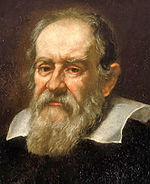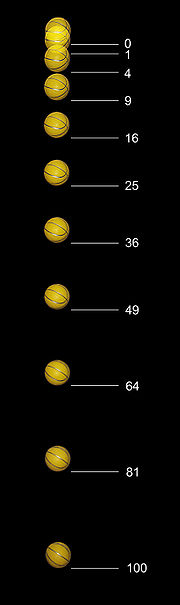Sometime prior to 1638, Galileo turned his attention to the phenomenon of objects falling under the influence of Earth’s gravity, and he was actively attempting to characterize these motions. Galileo was not the first to investigate Earth’s gravitational field, nor was he the first to accurately describe its fundamental characteristics. However, Galileo’s reliance on scientific experimentation to establish physical principles would have a profound effect on future generations of scientists. Galileo used a number of scientific experiments to characterize free fall motion. It is unclear if these were just hypothetical experiments used to illustrate a concept, or if they were real experiments performed by Galileo, but the results obtained from these experiments were both realistic and compelling. A biography by Galileo's pupil Vincenzo Viviani
Vincenzo Viviani
Vincenzo Viviani was an Italian mathematician and scientist. He was a pupil of Torricelli and a disciple of Galileo.-Biography:...

stated that Galileo had dropped ball
Ball
A ball is a round, usually spherical but sometimes ovoid, object with various uses. It is used in ball games, where the play of the game follows the state of the ball as it is hit, kicked or thrown by players. Balls can also be used for simpler activities, such as catch, marbles and juggling...

s of the same material, but different masses, from the Leaning Tower of Pisa
Leaning Tower of Pisa
The Leaning Tower of Pisa or simply the Tower of Pisa is the campanile, or freestanding bell tower, of the cathedral of the Italian city of Pisa...

to demonstrate that their time of descent was independent of their mass. In support of this conclusion, Galileo had advanced the following theoretical argument: He asked if two bodies of different masses and different rates of fall are tied by a string, does the combined system fall faster because it is now more massive, or does the lighter body in its slower fall hold back the heavier body? The only convincing resolution to this question is that all bodies must fall at the same rate.

A later experiment was described in Galileo’s Two New Sciences published in 1638. One of Galileo’s fictional characters, Salviati, describes an experiment using a bronze ball and a wooden ramp. The wooden ramp was "12 cubits long, half a cubit wide and three finger-breadths thick" with a straight, smooth, polished groove. The groove was lined with "parchment
Parchment
Parchment is a thin material made from calfskin, sheepskin or goatskin, often split. Its most common use was as a material for writing on, for documents, notes, or the pages of a book, codex or manuscript. It is distinct from leather in that parchment is limed but not tanned; therefore, it is very...

, also smooth and polished as possible". And into this groove was placed "a hard, smooth and very round bronze ball". The ramp was inclined at various angle
Angle
In geometry, an angle is the figure formed by two rays sharing a common endpoint, called the vertex of the angle.Angles are usually presumed to be in a Euclidean plane with the circle taken for standard with regard to direction. In fact, an angle is frequently viewed as a measure of an circular arc...

s to slow the acceleration enough so that the elapsed time could be measured. The ball was allowed to roll a known distance down the ramp, and the time taken for the ball to move the known distance was measured. The time was measured using a water clock described as follows:
"a large vessel of water placed in an elevated position; to the bottom of this vessel was soldered a pipe of small diameter giving a thin jet of water, which we collected in a small glass during the time of each descent, whether for the whole length of the channel or for a part of its length; the water thus collected was weighed, after each descent, on a very accurate balance; the differences and ratios of these weights gave us the differences and ratios of the times, and this with such accuracy that although the operation was repeated many, many times, there was no appreciable discrepancy in the results.".

Galileo found that for an object in free fall, the distance that the object has fallen is always proportional to the square of the elapsed time: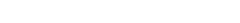Galileo Galilei died in Arcetri, Italy (near Florence), on 8 January 1642. Galileo had shown that objects in free fall under the influence of the Earth’s gravitational field have a constant acceleration, and Galileo’s contemporary, Johannes Kepler, had shown that the planets follow elliptical paths under the influence of the Sun’s gravitational mass. However, the relationship between Galileo’s gravitational field and Kepler’s gravitational mass wasn’t comprehended during Galileo’s lifetime.

### Newtonian gravitational mass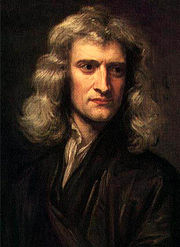Earth's Moon | Mass of Earth
Semi-major axis
Semi-major axis
The major axis of an ellipse is its longest diameter, a line that runs through the centre and both foci, its ends being at the widest points of the shape...

Sidereal orbital period
0.002 569 AU
Astronomical unit
An astronomical unit is a unit of length equal to about or approximately the mean Earth–Sun distance....

0.074 802 sidereal year
Sidereal year
A sidereal year is the time taken by the Earth to orbit the Sun once with respect to the fixed stars. Hence it is also the time taken for the Sun to return to the same position with respect to the fixed stars after apparently travelling once around the ecliptic. It was equal to at noon 1 January...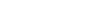=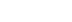Earth's Gravity Earth's Radius
0.009806656 375 km

Robert Hooke
Robert Hooke
Robert Hooke FRS was an English natural philosopher, architect and polymath.His adult life comprised three distinct periods: as a scientific inquirer lacking money; achieving great wealth and standing through his reputation for hard work and scrupulous honesty following the great fire of 1666, but...

published his concept of gravitational forces in 1674, stating that: “all Cœlestial Bodies whatsoever, have an attraction or gravitating power towards their own Centers" [and] "they do also attract all the other Cœlestial Bodies that are within the sphere of their activity”. He further states that gravitational attraction increases “by how much the nearer the body wrought upon is to their own center.” In a correspondence of 1679–1680 between Robert Hooke and Isaac Newton, Hooke conjectures that gravitational forces might decrease according to the square of the distance between the two bodies. Hooke urged Newton, who was a pioneer in the development of calculus
Calculus
Calculus is a branch of mathematics focused on limits, functions, derivatives, integrals, and infinite series. This subject constitutes a major part of modern mathematics education. It has two major branches, differential calculus and integral calculus, which are related by the fundamental theorem...

, to work through the mathematical details of Keplerian orbits to determine if Hooke’s hypothesis was correct. Newton’s own investigations verified that Hooke was correct, but due to personal differences between the two men, Newton chose not to reveal this to Hooke. Isaac Newton kept quiet about his discoveries until 1684, at which time he told a friend, Edmond Halley
Edmond Halley
Edmond Halley FRS was an English astronomer, geophysicist, mathematician, meteorologist, and physicist who is best known for computing the orbit of the eponymous Halley's Comet. He was the second Astronomer Royal in Britain, following in the footsteps of John Flamsteed.-Biography and career:Halley...

, that he had solved the problem of gravitational orbits, but had misplaced the solution in his office. After being encouraged by Halley, Newton decided to develop his ideas about gravity and publish all of his findings. In November of 1684, Isaac Newton sent a document to Edmund Halley, now lost but presumed to have been titled De motu corporum in gyrum
De motu corporum in gyrum
De motu corporum in gyrum is the title of a manuscript by Isaac Newton sent to Edmond Halley in November 1684...

(Latin: "On the motion of bodies in an orbit"). Halley presented Newton’s findings to the Royal Society
Royal Society
The Royal Society of London for Improving Natural Knowledge, known simply as the Royal Society, is a learned society for science, and is possibly the oldest such society in existence. Founded in November 1660, it was granted a Royal Charter by King Charles II as the "Royal Society of London"...

of London, with a promise that a fuller presentation would follow. Newton later recorded his ideas in a three book set, entitled Philosophiæ Naturalis Principia Mathematica  (Latin: "Mathematical Principles of Natural Philosophy"). The first was received by the Royal Society on 28 April 1685–6, the second on 2 March 1686–7, and the third on 6 April 1686–7. The Royal Society published Newton’s entire collection at their own expense in May of 1686–7.

Isaac Newton had bridged the gap between Kepler’s gravitational mass and Galileo’s gravitational acceleration, and proved the following relationship: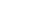,
where g is the apparent acceleration of a body as it passes through a region of space where gravitational fields exist, μ is the gravitational mass (standard gravitational parameter
Standard gravitational parameter
In astrodynamics, the standard gravitational parameter μ of a celestial body is the product of the gravitational constant G and the mass M of the body.\mu=GM \ The SI units of the standard gravitational parameter are m3s−2....

) of the body causing gravitational fields, and r is the radial coordinate (the distance between the centers of the two bodies).

By finding the exact relationship between a body's gravitational mass and its gravitational field, Newton provided a second method for measuring gravitational mass. The mass of the Earth can be determined using Kepler’s method (from the orbit of Earth’s Moon), or it can be determined by measuring the gravitational acceleration on the Earth’s surface, and multiplying that by the square of the Earth’s radius. The mass of the Earth is approximately three millionths of the mass of the Sun. To date, no other accurate method for measuring gravitational mass has been discovered.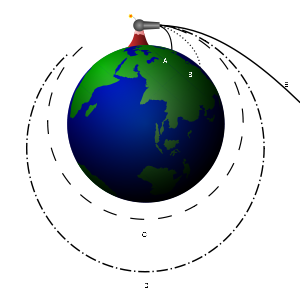#### Newton's cannonball

Newton's cannonball was a thought experiment
Thought experiment
A thought experiment or Gedankenexperiment considers some hypothesis, theory, or principle for the purpose of thinking through its consequences...

used to bridge the gap between Galileo’s gravitational acceleration and Kepler’s elliptical orbits. It appeared in Newton's 1728 book A Treatise of the System of the World. According to Galileo’s concept of gravitation, a dropped stone falls with constant acceleration down towards the Earth. However, Newton explains that when a stone is thrown horizontally (meaning sideways or perpendicular to Earth’s gravity) it follows a curved path. “For a stone projected is by the pressure of its own weight forced out of the rectilinear path, which by the projection alone it should have pursued, and made to describe a curve line in the air; and through that crooked way is at last brought down to the ground. And the greater the velocity is with which it is projected, the farther it goes before it falls to the Earth.”

Newton further reasons that if an object were “projected in an horizontal direction from the top of a high mountain” with sufficient velocity, “it would reach at last quite beyond the circumference of the Earth, and return to the mountain from which it was projected.” Newton’s thought experiment is illustrated in the image to the right. A cannon on top of a very high mountain shoots a cannon ball in a horizontal direction. If the speed is low, it simply falls back on Earth (paths A and B). However, if the speed is equal to or higher than some threshold (orbital velocity
Orbital speed
The orbital speed of a body, generally a planet, a natural satellite, an artificial satellite, or a multiple star, is the speed at which it orbits around the barycenter of a system, usually around a more massive body...

), but not high enough to leave Earth altogether (escape velocity
Escape velocity
In physics, escape velocity is the speed at which the kinetic energy plus the gravitational potential energy of an object is zero gravitational potential energy is negative since gravity is an attractive force and the potential is defined to be zero at infinity...

), it will continue revolving around Earth along an elliptical orbit (C and D).

#### Universal gravitational mass and amount

Newton's cannonball illustrated the relationship between the Earth’s gravitational mass and its gravitational field; however, a number of other ambiguities still remained. Robert Hooke had asserted in 1674 that: "all Celestial Bodies whatsoever, have an attraction or gravitating power towards their own Centers", but Hooke had neither explained why this gravitating attraction was unique to celestial bodies, nor had he explained why the attraction was directed towards the center of a celestial body.
To answer these questions, Newton introduced the entirely new concept that gravitational mass is “universal”: meaning that every object has gravitational mass, and therefore, every object generates a gravitational field. Newton further assumed that the strength of each object’s gravitational field would decrease according to the square of the distance to that object. With these assumptions in mind, Newton calculated what the overall gravitational field would be if a large collection of small objects were formed into a giant spherical body. Newton found that a giant spherical body (like the Earth or Sun, with roughly uniform density at each given radius), would have a gravitational field which was proportional to the total mass of the body, and inversely proportional to the square of the distance to the body’s center.

Newton's concept of universal gravitational mass is illustrated in the image to the left. Every piece of the Earth has gravitational mass and every piece creates a gravitational field directed towards that piece. However, the overall effect of these many fields is equivalent to a single powerful field directed towards the center of the Earth. The apple behaves as if a single powerful gravitational field were accelerating it towards the Earth’s center.

Newton’s concept of universal gravitational mass puts gravitational mass on an equal footing with the traditional concepts of weight and amount. For example, the ancient Romans had used the carob seed as a weight standard. The Romans could place an object with an unknown weight on one side of a balance scale and place carob seeds on the other side of the scale, increasing the number of seeds until the scale was balanced. If an object’s weight was equivalent to 1728 carob seeds, then the object was said to weigh one Roman pound.

According to Newton’s theory of universal gravitation, each carob seed produces gravitational fields. Therefore, if one were to gather an immense number of carob seeds and form them into an enormous sphere, then the gravitational field of the sphere would be proportional to the number of carob seeds in the sphere. Hence, it should be theoretically possible to determine the exact number of carob seeds that would be required to produce a gravitational field similar to that of the Earth or Sun. And since the Roman weight units were all defined in terms of carob seeds, then knowing the Earth’s, or Sun's “carob seed mass” would allow one to calculate the mass in Roman pounds, or Roman ounces, or any other Roman unit.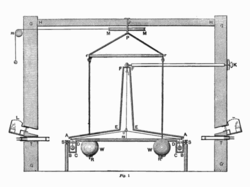This possibility extends beyond Roman units and the carob seed. The British avoirdupois pound, for example, was originally defined to be equal to 7,000 barley grains. Therefore, if one could determine the Earth’s “barley grain mass” (the number of barley grains required to produce a gravitational field similar to that of the Earth), then this would allow one to calculate the Earth’s mass in avoirdupois pounds. Also, the original kilogram was defined to be equal in mass to a liter of pure water (the modern kilogram is defined by the man-made international prototype kilogram). Thus, the mass of the Earth in kilograms could theoretically be determined by ascertaining how many liters of pure water (or international prototype kilograms) would be required to produce gravitational fields similar to those of the Earth. In fact, it is a simple matter of abstraction to realize that any traditional mass unit can theoretically be used to measure gravitational mass.

Measuring gravitational mass in terms of traditional mass units is simple in principle, but extremely difficult in practice. According to Newton’s theory all objects produce gravitational fields and it is theoretically possible to collect an immense number of small objects and form them into an enormous gravitating sphere. However, from a practical standpoint, the gravitational fields of small objects are extremely weak and difficult to measure. And if one were to collect an immense number of objects, the resulting sphere would probably be too large to construct on the surface of the Earth, and too expensive to construct in space. Newton’s books on universal gravitation were published in the 1680s, but the first successful measurement of the Earth’s mass in terms of traditional mass units, the Cavendish experiment
Cavendish experiment
The Cavendish experiment, performed in 1797–98 by British scientist Henry Cavendish was the first experiment to measure the force of gravity between masses in the laboratory, and the first to yield accurate values for the gravitational constant. Because of the unit conventions then in use,...

, didn’t occur until 1797, over a hundred years later. Cavendish found that the Earth's density was 5.448 ± 0.033 times that of water. As of 2009, the Earth’s mass in kilograms is only known to around five digits of accuracy, whereas its gravitational mass is known to over significant figures.

## Inertial and gravitational mass

Although inertial mass, passive gravitational mass and active gravitational mass are conceptually distinct, no experiment has ever unambiguously demonstrated any difference between them. In classical mechanics
Classical mechanics
In physics, classical mechanics is one of the two major sub-fields of mechanics, which is concerned with the set of physical laws describing the motion of bodies under the action of a system of forces...

, Newton's third law implies that active and passive gravitational mass must always be identical (or at least proportional), but the classical theory offers no compelling reason why the gravitational mass has to equal the inertial mass. That it does is merely an empirical fact.

Albert Einstein
Albert Einstein
Albert Einstein was a German-born theoretical physicist who developed the theory of general relativity, effecting a revolution in physics. For this achievement, Einstein is often regarded as the father of modern physics and one of the most prolific intellects in human history...

developed his general theory of relativity starting from the assumption that this correspondence between inertial and (passive) gravitational mass is not accidental: that no experiment will ever detect a difference between them (the weak version of the equivalence principle
Equivalence principle
In the physics of general relativity, the equivalence principle is any of several related concepts dealing with the equivalence of gravitational and inertial mass, and to Albert Einstein's assertion that the gravitational "force" as experienced locally while standing on a massive body is actually...

). However, in the resulting theory, gravitation is not a force and thus not subject to Newton's third law, so "the equality of inertial and active gravitational mass [...] remains as puzzling as ever".

### Inertial mass

Inertial mass is the mass of an object measured by its resistance to acceleration.

To understand what the inertial mass of a body is, one begins with classical mechanics
Classical mechanics
In physics, classical mechanics is one of the two major sub-fields of mechanics, which is concerned with the set of physical laws describing the motion of bodies under the action of a system of forces...

and Newton's Laws of Motion
Newton's laws of motion
Newton's laws of motion are three physical laws that form the basis for classical mechanics. They describe the relationship between the forces acting on a body and its motion due to those forces...

. Later on, we will see how our classical definition of mass must be altered if we take into consideration the theory of special relativity
Special relativity
Special relativity is the physical theory of measurement in an inertial frame of reference proposed in 1905 by Albert Einstein in the paper "On the Electrodynamics of Moving Bodies".It generalizes Galileo's...

, which is more accurate than classical mechanics. However, the implications of special relativity will not change the meaning of "mass" in any essential way.

According to Newton's second law
Newton's laws of motion
Newton's laws of motion are three physical laws that form the basis for classical mechanics. They describe the relationship between the forces acting on a body and its motion due to those forces...

, we say that a body has a mass m if, at any instant of time, it obeys the equation of motion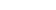,

where F is the force
Force
In physics, a force is any influence that causes an object to undergo a change in speed, a change in direction, or a change in shape. In other words, a force is that which can cause an object with mass to change its velocity , i.e., to accelerate, or which can cause a flexible object to deform...

acting on the body and α is the acceleration
Acceleration
In physics, acceleration is the rate of change of velocity with time. In one dimension, acceleration is the rate at which something speeds up or slows down. However, since velocity is a vector, acceleration describes the rate of change of both the magnitude and the direction of velocity. ...

of the body.Newton's second law is valid only for bodies of constant mass. For the moment, we will put aside the question of what "force acting on the body" actually means.

This equation illustrates how mass relates to the inertia of a body. Consider two objects with different masses. If we apply an identical force to each, the object with a bigger mass will experience a smaller acceleration, and the object with a smaller mass will experience a bigger acceleration. We might say that the larger mass exerts a greater "resistance" to changing its state of motion in response to the force.

However, this notion of applying "identical" forces to different objects brings us back to the fact that we have not really defined what a force is. We can sidestep this difficulty with the help of Newton's third law, which states that if one object exerts a force on a second object, it will experience an equal and opposite force. To be precise, suppose we have two objects A and B, with constant inertial masses mX and mY. We isolate the two objects from all other physical influences, so that the only forces present are the force exerted on X by Y, which we denote FXY, and the force exerted on Y by X, which we denote FYX. Newton's second law states that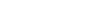,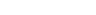,
where αX and αY are the accelerations of X and Y, respectively. Suppose that these accelerations are non-zero, so that the forces between the two objects are non-zero. This occurs, for example, if the two objects are in the process of colliding with one another. Newton's third law then states that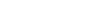;
and thus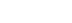.

Note that our requirement that αX be non-zero ensures that the fraction is well-defined.

This is, in principle, how we would measure the inertial mass of an object. We choose a "reference" object and define its mass mY as (say) 1 kilogram. Then we can measure the mass of any other object in the universe by colliding it with the reference object and measuring the accelerations.

### Newtonian gravitational mass

The Newtonian concept of gravitational mass rests on Newton's law of gravitation
Newton's law of universal gravitation
Newton's law of universal gravitation states that every point mass in the universe attracts every other point mass with a force that is directly proportional to the product of their masses and inversely proportional to the square of the distance between them...

. Let us suppose we have two objects A and B, separated by a distance rAB. The law of gravitation states that if A and B have gravitational masses MA and MB respectively, then each object exerts a gravitational force on the other, of magnitude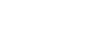,
where G is the universal gravitational constant
Gravitational constant
The gravitational constant, denoted G, is an empirical physical constant involved in the calculation of the gravitational attraction between objects with mass. It appears in Newton's law of universal gravitation and in Einstein's theory of general relativity. It is also known as the universal...

. The above statement may be reformulated in the following way: if g is the magnitude at a given location in a gravitational field, then the gravitational force on an object with gravitational mass M is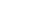.
This is the basis by which masses are determined by weighing. In simple spring scales, for example, the force F is proportional to the displacement of the spring
Spring (device)
A spring is an elastic object used to store mechanical energy. Springs are usually made out of spring steel. Small springs can be wound from pre-hardened stock, while larger ones are made from annealed steel and hardened after fabrication...

beneath the weighing pan, as per Hooke's law
Hooke's law
In mechanics, and physics, Hooke's law of elasticity is an approximation that states that the extension of a spring is in direct proportion with the load applied to it. Many materials obey this law as long as the load does not exceed the material's elastic limit. Materials for which Hooke's law...

, and the scales are calibrated
Calibration
Calibration is a comparison between measurements – one of known magnitude or correctness made or set with one device and another measurement made in as similar a way as possible with a second device....

to take g into account, allowing the mass M to be read off. A balance measures gravitational mass; only the spring scale measures weight.

### Equivalence of inertial and gravitational masses

The equivalence of inertial and gravitational masses is sometimes referred to as the Galilean equivalence principle or weak equivalence principle. The most important consequence of this equivalence principle applies to freely falling objects. Suppose we have an object with inertial and gravitational masses m and M respectively. If the only force acting on the object comes from a gravitational field g, combining Newton's second law and the gravitational law yields the acceleration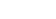This says that the ratio of gravitational to inertial mass of any object is equal to some constant K if and only if
If and only if
In logic and related fields such as mathematics and philosophy, if and only if is a biconditional logical connective between statements....

all objects fall at the same rate in a given gravitational field. This phenomenon is referred to as the 'universality of free-fall'. (In addition, the constant K can be taken to be 1 by defining our units appropriately.)

The first experiments demonstrating the universality of free-fall were conducted by Galileo
Galileo Galilei
Galileo Galilei , was an Italian physicist, mathematician, astronomer, and philosopher who played a major role in the Scientific Revolution. His achievements include improvements to the telescope and consequent astronomical observations and support for Copernicanism...

. It is commonly stated that Galileo obtained his results by dropping objects from the Leaning Tower of Pisa
Leaning Tower of Pisa
The Leaning Tower of Pisa or simply the Tower of Pisa is the campanile, or freestanding bell tower, of the cathedral of the Italian city of Pisa...

, but this is most likely apocryphal; actually, he performed his experiments with balls rolling down inclined plane
Inclined plane
The inclined plane is one of the original six simple machines; as the name suggests, it is a flat surface whose endpoints are at different heights. By moving an object up an inclined plane rather than completely vertical, the amount of force required is reduced, at the expense of increasing the...

s. Increasingly precise experiments have been performed, such as those performed by Loránd Eötvös
Loránd Eötvös
Baron Loránd Eötvös de Vásárosnamény , more commonly called Baron Roland von Eötvös in English literature, was a Hungarian physicist. He is remembered today largely for his work on gravitation and surface tension.-Life:...

, using the torsion balance pendulum, in 1889. , no deviation from universality, and thus from Galilean equivalence, has ever been found, at least to the precision 10−12. More precise experimental efforts are still being carried out.

The universality of free-fall only applies to systems in which gravity is the only acting force. All other forces, especially friction
Friction
Friction is the force resisting the relative motion of solid surfaces, fluid layers, and/or material elements sliding against each other. There are several types of friction:...

and air resistance, must be absent or at least negligible
Negligible
Negligible refers to the quantities so small that they can be ignored when studying the larger effect. Although related to the more mathematical concepts of infinitesimal, the idea of negligibility is particularly useful in practical disciplines like physics, chemistry, mechanical and electronic...

. For example, if a hammer and a feather are dropped from the same height through the air on Earth, the feather will take much longer to reach the ground; the feather is not really in free-fall because the force of air resistance upwards against the feather is comparable to the downward force of gravity. On the other hand, if the experiment is performed in a vacuum
Vacuum
In everyday usage, vacuum is a volume of space that is essentially empty of matter, such that its gaseous pressure is much less than atmospheric pressure. The word comes from the Latin term for "empty". A perfect vacuum would be one with no particles in it at all, which is impossible to achieve in...

, in which there is no air resistance, the hammer and the feather should hit the ground at exactly the same time (assuming the acceleration of both objects towards each other, and of the ground towards both objects, for its own part, is negligible). This can easily be done in a high school laboratory by dropping the objects in transparent tubes that have the air removed with a vacuum pump. It is even more dramatic when done in an environment that naturally has a vacuum, as David Scott
David Scott
David Randolph Scott is an American engineer, test pilot, retired U.S. Air Force officer, and former NASA astronaut and engineer, who was one of the third group of astronauts selected by NASA in October 1963...

did on the surface of the Moon
Moon
The Moon is Earth's only known natural satellite,There are a number of near-Earth asteroids including 3753 Cruithne that are co-orbital with Earth: their orbits bring them close to Earth for periods of time but then alter in the long term . These are quasi-satellites and not true moons. For more...

during Apollo 15
Apollo 15
Apollo 15 was the ninth manned mission in the American Apollo space program, the fourth to land on the Moon and the eighth successful manned mission. It was the first of what were termed "J missions", long duration stays on the Moon with a greater focus on science than had been possible on previous...

.

A stronger version of the equivalence principle, known as the Einstein equivalence principle or the strong equivalence principle, lies at the heart of the general theory of relativity
General relativity
General relativity or the general theory of relativity is the geometric theory of gravitation published by Albert Einstein in 1916. It is the current description of gravitation in modern physics...

. Einstein's equivalence principle states that within sufficiently small regions of space-time, it is impossible to distinguish between a uniform acceleration and a uniform gravitational field. Thus, the theory postulates that the force acting on a massive object caused by a gravitational field is a result of the object's tendency to move in a straght line (in other words its inertia) and should therefore be a function of its inertial mass and the strength of the gravitational field.

## Mass and energy in special relativity

The term mass in special relativity
Special relativity
Special relativity is the physical theory of measurement in an inertial frame of reference proposed in 1905 by Albert Einstein in the paper "On the Electrodynamics of Moving Bodies".It generalizes Galileo's...

usually refers to the rest mass of the object, which is the Newtonian mass as measured by an observer moving along with the object. The invariant mass
Invariant mass
The invariant mass, rest mass, intrinsic mass, proper mass or just mass is a characteristic of the total energy and momentum of an object or a system of objects that is the same in all frames of reference related by Lorentz transformations...

is another name for the rest mass of single particles. However, the more general invariant mass (calculated with a more complicated formula) may also be applied to systems of particles in relative motion, and because of this, is usually reserved for systems which consist of widely separated high-energy particles. The invariant mass of systems is the same for all observers and inertial frames, and cannot be destroyed, and is thus conserved, so long as the system is closed. In this case, "closure" implies that an idealized boundary is drawn around the system, and no mass/energy is allowed across it.

In as much as energy
Energy
In physics, energy is an indirectly observed quantity. It is often understood as the ability a physical system has to do work on other physical systems...

is conserved in closed systems in relativity, the mass of a system is also a quantity which is conserved: this means it does not change over time, even as some types of particles are converted to others. For any given observer, the mass of any system is separately conserved and cannot change over time, just as energy is separately conserved and cannot change over time. The incorrect popular idea that mass may be converted to (massless) energy in relativity is because some matter
Matter
Matter is a general term for the substance of which all physical objects consist. Typically, matter includes atoms and other particles which have mass. A common way of defining matter is as anything that has mass and occupies volume...

particles may in some cases be converted to types of energy which are not matter (such as light, kinetic energy, and the potential energy in magnetic, electric, and other fields). However, this confuses "matter" (a non-conserved and ill-defined thing) with mass (which is well-defined and is conserved). Even if not considered "matter," all types of energy still continue to exhibit mass in relativity. Thus, mass and energy do not change into one another in relativity; rather, both are names for the same thing, and neither mass nor energy appear without the other. "Matter" particles may not be conserved in reactions in relativity, but closed-system mass always is.

For example, a nuclear bomb in an idealized super-strong box, sitting on a scale, would in theory show no change in mass when detonated (although the inside of the box would become much hotter). In such a system, the mass of the box would change only if energy were allowed to escape from the box as light or heat. However, in that case, the removed energy would take its associated mass with it. Letting heat out of such a system is simply a way to remove mass. Thus, mass, like energy, cannot be destroyed, but only moved from one place to another.

In bound systems, the binding energy
Binding energy
Binding energy is the mechanical energy required to disassemble a whole into separate parts. A bound system typically has a lower potential energy than its constituent parts; this is what keeps the system together—often this means that energy is released upon the creation of a bound state...

must (often) be subtracted from the mass of the unbound system, simply because this energy has mass, and this mass is subtracted from the system when it is given off, at the time it is bound. Mass is not conserved in this process because the system is not closed during the binding process. A familiar example is the binding energy of atomic nuclei, which appears as other types of energy (such as gamma rays) when the nuclei are formed, and (after being given off) results in nuclide
Nuclide
A nuclide is an atomic species characterized by the specific constitution of its nucleus, i.e., by its number of protons Z, its number of neutrons N, and its nuclear energy state....

s which have less mass than the free particles (nucleon
Nucleon
In physics, a nucleon is a collective name for two particles: the neutron and the proton. These are the two constituents of the atomic nucleus. Until the 1960s, the nucleons were thought to be elementary particles...

s) of which they are composed.

The term relativistic mass is also used, and this is the total quantity of energy in a body or system (divided by c
Celeritas
Celeritas is a Latin word, translated as "swiftness" or "speed". It is often given as the origin of the symbol c, the universal notation for the speed of light in a vacuum, as popularized in Albert Einstein's famous equation E = mc². In SI units, the speed of light in a vacuum is defined as...

2). The relativistic mass (of a body or system of bodies) includes a contribution from the kinetic energy of the body, and is larger the faster the body moves, so unlike the invariant mass, the relativistic mass depends on the observer's frame of reference
Frame of reference
A frame of reference in physics, may refer to a coordinate system or set of axes within which to measure the position, orientation, and other properties of objects in it, or it may refer to an observational reference frame tied to the state of motion of an observer.It may also refer to both an...

. However, for given single frames of reference and for closed systems, the relativistic mass is also a conserved quantity.

Because the relativistic mass is proportional to the energy, it has gradually fallen into disuse among physicists. There is disagreement over whether the concept remains pedagogically useful.

For a discussion of mass in general relativity
General relativity
General relativity or the general theory of relativity is the geometric theory of gravitation published by Albert Einstein in 1916. It is the current description of gravitation in modern physics...

, see mass in general relativity
Mass in General Relativity
The concept of mass in general relativity is more complex than the concept of mass in special relativity. In fact, general relativity does not offer a single definition for the term mass, but offers several different definitions which are applicable under different circumstances...

.

## External links

The source of this article is wikipedia, the free encyclopedia.  The text of this article is licensed under the GFDL.